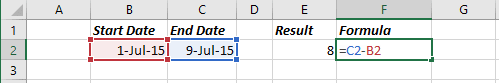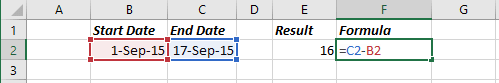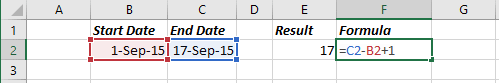# Calculating the number of days between two dates

Excel
If you need to count the number of dates between two dates in Excel, you don't need to use DATEDIF and other Excel date manipulation functions. Date in Excel isn't a separate type, but a number with proper formatting. The integer part of this number represents days since the fixed moment for all dates. Subtract one date from another, and you will get the number of days between two dates. Also, you can add or subtract an integer from the date, and get the date in the future or the past.

For example, you have a financial worksheet that calculates interest earned on saving at the deposit account. If your spreadsheet contains the open date and the close date for the account, you can calculate the number of days the account was open:

= C2 - B2Excel automatically formats this formula cell as a date rather than a numeric value. Therefore, you need to switch to the number format to see the difference as a number. If cell B2 contains a more recent date than the date in cell C2, the result is negative.

Sometimes, calculating the difference between the two days is more complicated. For example, you start a trip on September 1 and return on September 17. Subtracting September 1 from September 17 produces a trip duration of 16 days:However, dates difference doesn't take into account the start and the end date, and the actual duration is 17 days:

= C2 - B2 + 1See also this tip in French: Calculer le nombre de jours entre deux dates.